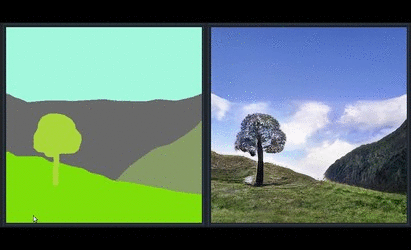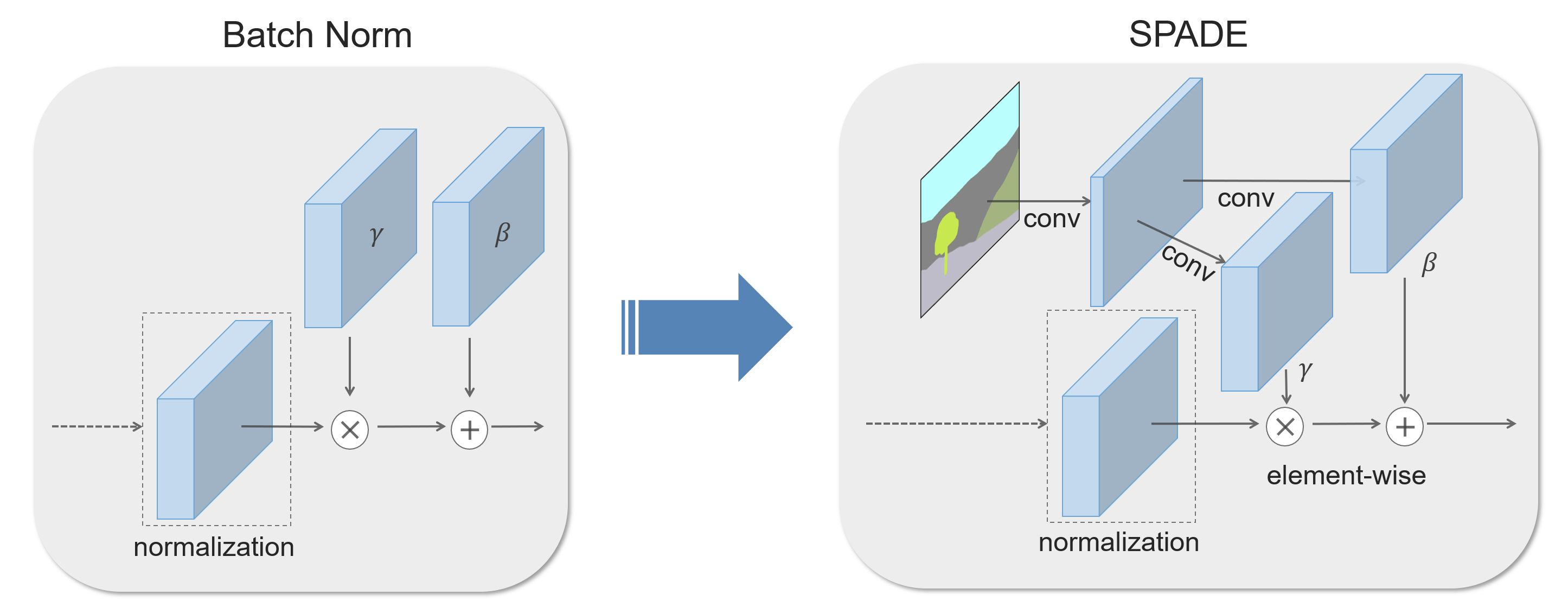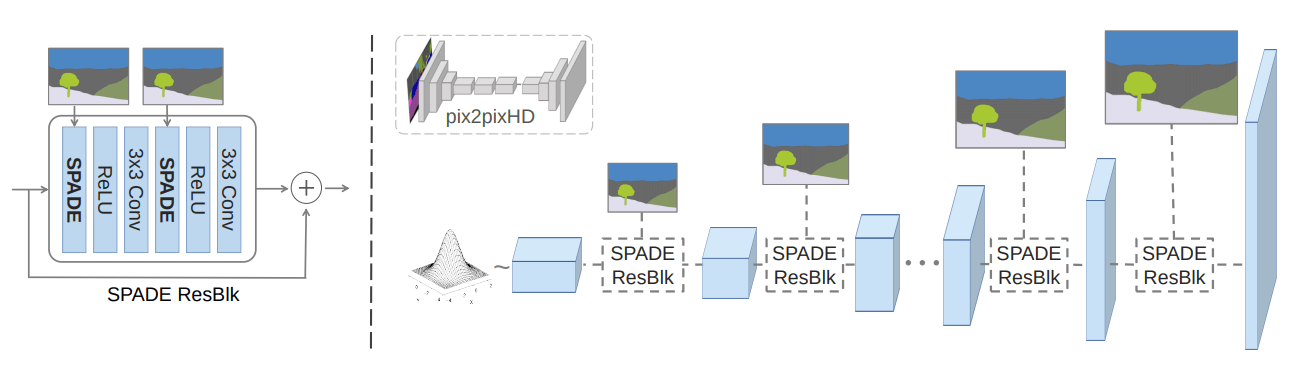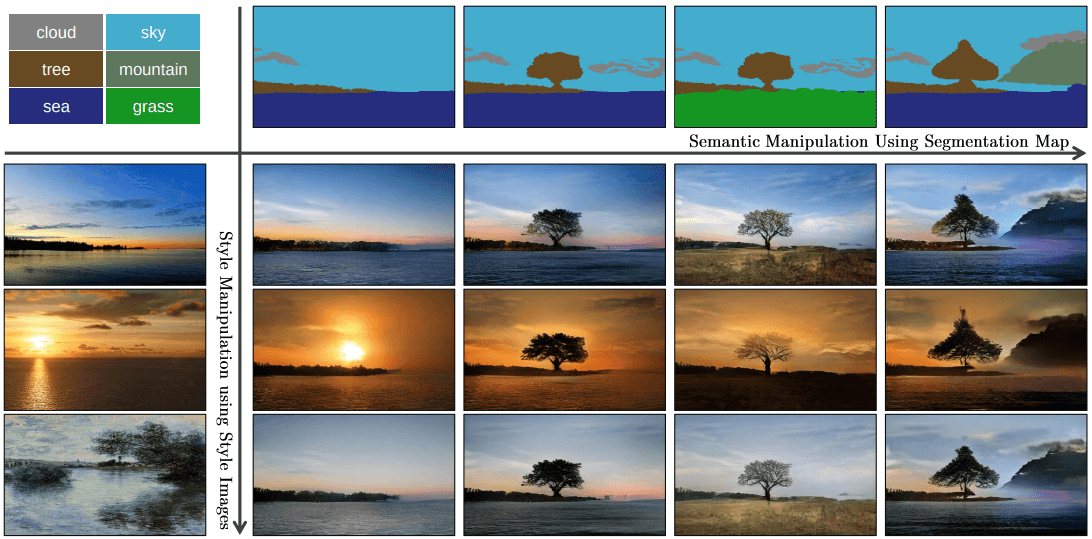## Intro

### Normalization layers

Conditional 方式有 Conditional BNAdaIN ， 它们先将数据调整为 0 均值和 1 方差，然后使用额外数据学到的仿射变化进一步调整数据。

## Semantic Image SynthesisSPADE 规范化的数学表述为

$\mu^i_c$ 和 $\sigma^i_c$ 是对通道上的数据计算的实数，而 $\gamma^i_{c,y,x}$ 和 $\beta^i_{c,y,x}$ 是对通道上数据卷积出来的一个相同大小 ($H\times W$) 的 tensor， 即对 $H\times W$ 空间上每个位置 ($x,y​$) 都有一个对应的值，所以该 denormalizationspatially0%